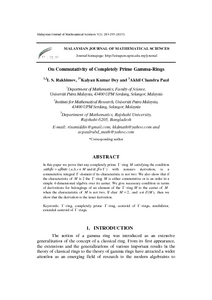# On commutativity of completely prime gamma-rings

## Citation

Rakhimov, Isamiddin Sattarovich and Dey, Kalyan Kumar and Paul, Akhil Chandra (2013) On commutativity of completely prime gamma-rings. Malaysian Journal of Mathematical Sciences, 7 (2). pp. 283-295. ISSN 1823-8343

## Abstract

In this paper we prove that any completely prime Γ -ring M satisfying the condition aαbβc=aβbαc = ( a,b,c ∈ M and α, β∈Γ )with nonzero derivation, is a commutative integral Γ -domain if its characteristic is not two. We also show that if the characteristic of M is 2 the Γ -ring M is either commutative or is an order in a simple 4-dimensional algebra over its center. We give necessary condition in terms of derivations for belongings of an element of the Γ -ring M to the center of M when the characteristic of M is not two. If char M = 2, and a Z M ∉ ( ), then we show that the derivation is the inner derivation.Preview
PDF
30157.pdfView Item